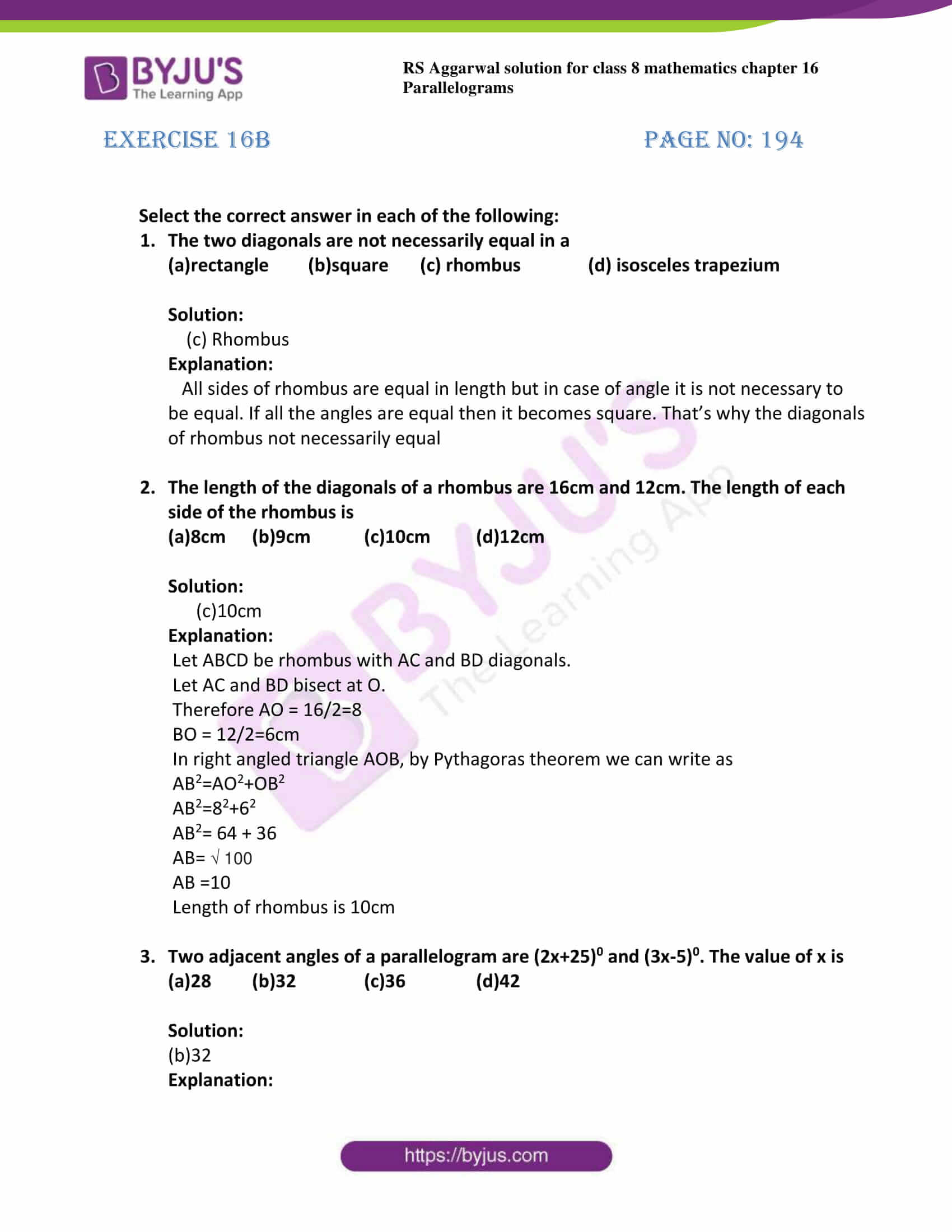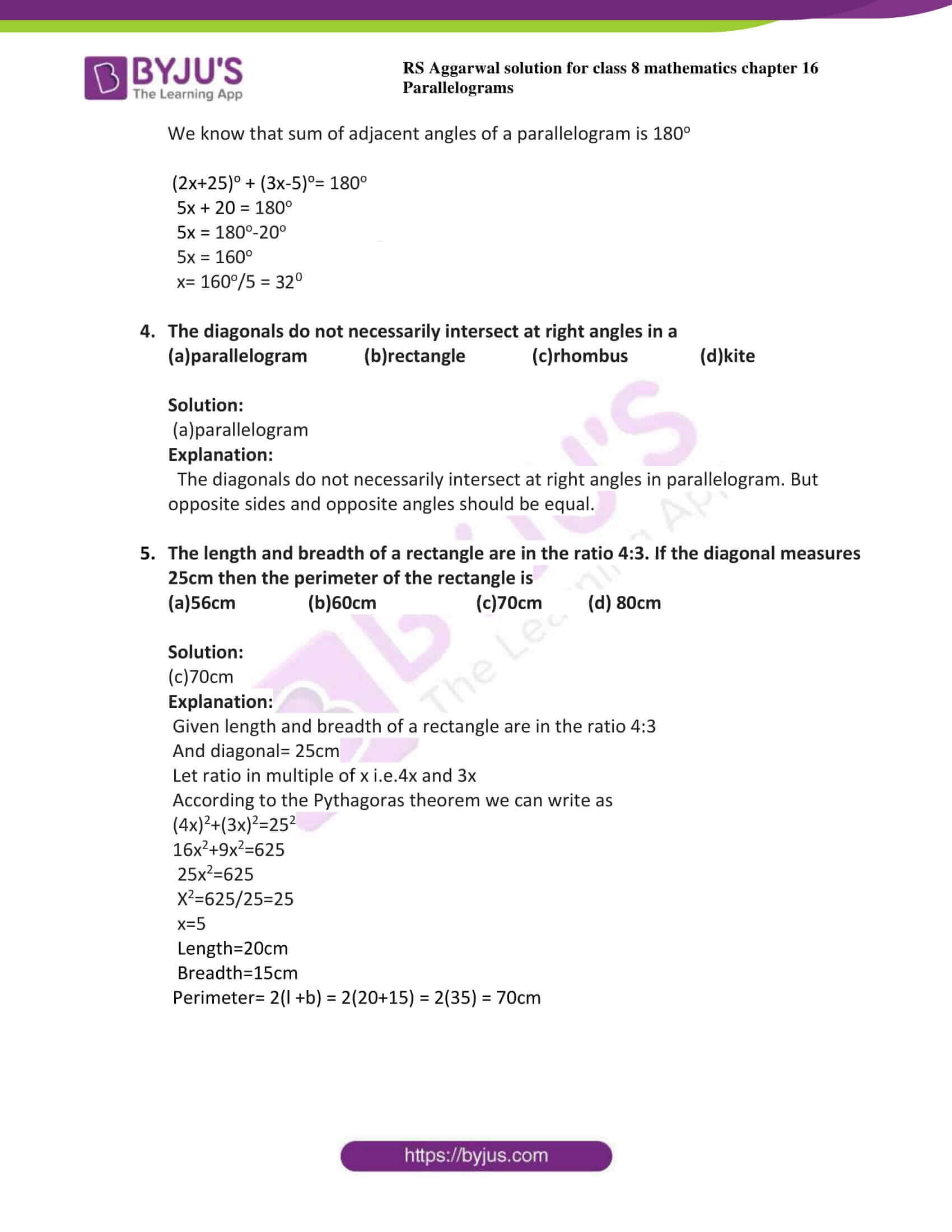# RS Aggarwal Solutions for Class 8 Maths Chapter 16 - Parallelograms Exercise 16B

The pdf of RS Aggarwal Solutions for the Exercise 16B of Class 8 Maths Chapter 16, Parallelogram are available here. BYJU’S experts in Maths have solved the questions present in this exercise. As this is the last exercise of this chapter, it contains multiple-choice questions. And will, in turn, help the student to get clarity about the topic. By practising the RS Aggarwal Solutions for class 8, students will be able to grasp the concepts correctly.

## Download PDF of RS Aggarwal Solutions for Class 8 Maths Chapter 16 – Parallelograms Exercise 16B### Access answers to Maths RS Aggarwal Solutions for Class 8 Chapter 16 – Parallelograms Exercise 16B

Select the correct answer in each of the following:

1. The two diagonals are not necessarily equal in a

(a)rectangle (b)square (c) rhombus (d) isosceles trapezium

Solution:

(c) Rhombus

Explanation:

All sides of rhombus are equal in length but in case of angle it is not necessary to be equal. If all the angles are equal then it becomes square. That’s why the diagonals of rhombus not necessarily equal

2. The length of the diagonals of a rhombus are 16cm and 12cm. The length of each side of the rhombus is

(a)8cm (b)9cm (c)10cm (d)12cm

Solution:

(c)10cm

Explanation:

Let ABCD be rhombus with AC and BD diagonals.

Let AC and BD bisect at O.

Therefore AO = 16/2=8

BO = 12/2=6cm

In right angled triangle AOB, by Pythagoras theorem we can write as

AB2=AO2+OB2

AB2=82+62

AB2= 64 + 36

AB= √ 100

AB =10

Length of rhombus is 10cm

3. Two adjacent angles of a parallelogram are (2x+25)0 and (3x-5)0. The value of x is

(a)28 (b)32 (c)36 (d)42

Solution:

(b)32

Explanation:

We know that sum of adjacent angles of a parallelogram is 180o

(2x+25)o + (3x-5)o= 180o

5x + 20 = 180o

5x = 180o-20o

5x = 160o

x= 160o/5 = 320

4. The diagonals do not necessarily intersect at right angles in a

(a)parallelogram (b)rectangle (c)rhombus (d)kite

Solution:

(a)parallelogram

Explanation:

The diagonals do not necessarily intersect at right angles in parallelogram. But opposite sides and opposite angles should be equal.

5. The length and breadth of a rectangle are in the ratio 4:3. If the diagonal measures 25cm then the perimeter of the rectangle is

(a)56cm (b)60cm (c)70cm (d) 80cm

Solution:

(c)70cm

Explanation:

Given length and breadth of a rectangle are in the ratio 4:3

And diagonal= 25cm

Let ratio in multiple of x i.e.4x and 3x

According to the Pythagoras theorem we can write as

(4x)2+(3x)2=252

16x2+9x2=625

25x2=625

X2=625/25=25

x=5

Length=20cm

Perimeter= 2(l +b) = 2(20+15) = 2(35) = 70cm

## RS Aggarwal Solutions for Class 8 Maths Chapter 16 – Parallelograms Exercise 16B

Exercise 16B of RS Aggarwal Solutions for Chapter 16, Parallelograms deals with the topics related to properties of quadrilaterals. Some of the topics focused prior to this exercise include the following.

• Properties of a parallelogram
• Diagonal properties of a rectangle
• Diagonal properties of a rhombus
• Diagonal properties of a square

The RS Aggarwal Solutions can help the students in practising and learning each and every concept as it provides solutions to all questions asked in the RS Aggarwal textbook. Those who aim to score high in the Maths of class 8 are advised to practice all the questions present in RS Aggarwal as many times as possible.